# 8 Clustering Methods From Scikit Learn We Should Know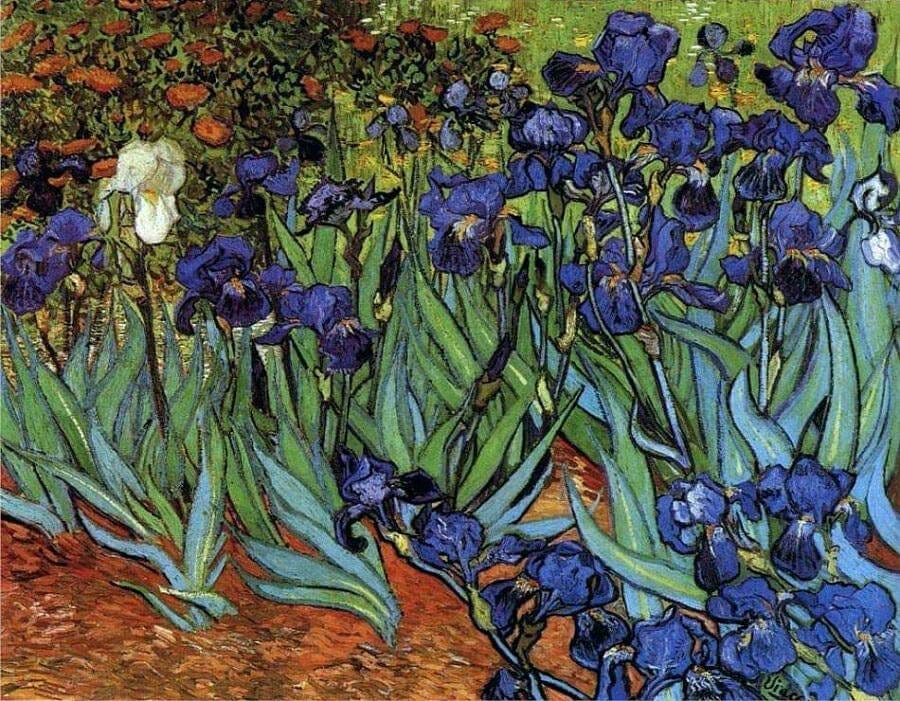Courtesy of www.VincentVanGogh.org

Scikit Learn is an open source, Python based very popular machine learning library. It supports various supervised (regression and classification) and unsupervised learning models.

In this blog, we’ll use 8 clustering methods or unsupervised machine learning models on the Iris Plants database (download from here and for details, refer here). Instead of going deep into the algorithms or mathematical details, we have limited our discussion on using the Scikit Learn clustering methods only.

The database contains the following details:

`1. Sepal Length in cm2. Sepal Width in cm3. Petal Length in cm4. Petal Width in cm5. Class: Iris Setosa or Iris Versicolour or Iris Virginica`

Let’s load the data and use it as a Spark table…

`df = spark.table ('iris_data_set')print(f"""There are {df.count()} records in the dataset.""")labelCol = "Class"df.show(5)`

…and convert the Spark DataFrame into a Panda DataFrame:

`import pandas as pddataset = df.toPandas()`

# Data Analysis

## Mean & Standard Deviation

If we want to have a quick look about how the data are distributed around the mean, we can describe the dataset:

## Scatter Matrix

If we see the correlation among the features we can see Petal_Length and Petal_Width have high positive correlation in between themselves (highlighted below).

Here, we’ll take these two features only to cluster the input dataset using the following methods.

# 1. K-means Clustering

The K-Means clustering partitions n observations into k clusters such that each observation belongs to the cluster with the nearest mean which is called the cluster centroid or center of the cluster. This algorithm tries to minimize the sum of distances between the points and their respective cluster centroid.

In this algorithm we have to pass the number of clusters k.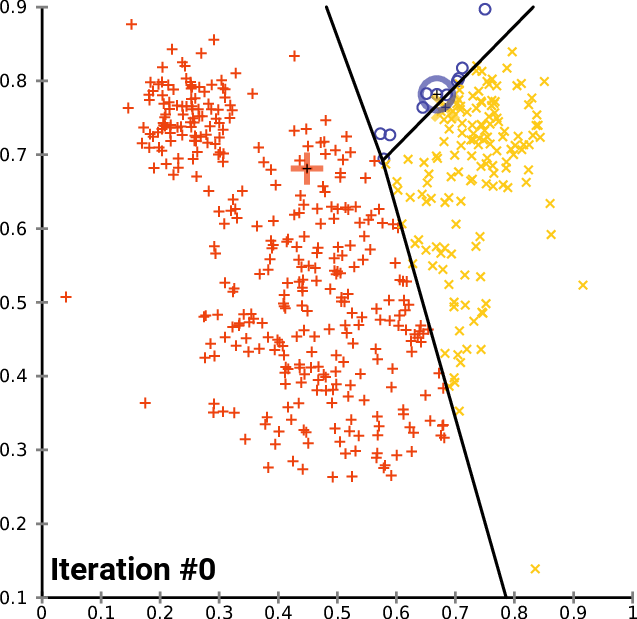Image source: Wikipedia

## Elbow Method

We can use the “elbow” method to find the optimal number of clusters required as an input for this algorithm. Here, we calculate the inertia (kmeans.inertia_) which is the sum of squared distances of samples to their closest cluster center for different proposed number of clusters. When these are plotted, it looks like an arm and the “elbow” point is the best value of k.

`import numpy as npimport matplotlib.pyplot as pltfrom sklearn.cluster import KMeanssum_sqrd = []for i in range(1, 11):    kmeans = KMeans(n_clusters = i, \                    init = 'k-means++', \                    random_state = 42)    kmeans.fit(X)    sum_sqrd.append(kmeans.inertia_) # inertia_: Sum of squared distances of samples to their closest cluster center.plt.plot(range(1, 11), sum_sqrd)plt.title('K-Means - the Elbow method')plt.xlabel('Number of clusters')plt.ylabel('Sum of squared distances')plt.show()`

Training the K-Means model on the dataset:

`kmeans = KMeans(n_clusters = 3, \                init = 'k-means++', \                random_state = 42)y_kmeans = kmeans.fit_predict(X)`

k-means++: we have used ‘k-means++’ as a method for initialization rather than ‘random’. These algorithms are used to choose initial values for K-means clustering. k-means++ uses a smarter way to initialize the centroids for better clustering.

Visualizing the clusters:

`# Visualising the clustersplt.figure(figsize=(12,8))plt.scatter(X[y_kmeans == 0, 0], X[y_kmeans == 0, 1], \            s = 100, c = 'red', label = 'Cluster 1')plt.scatter(X[y_kmeans == 1, 0], X[y_kmeans == 1, 1], \            s = 100, c = 'blue', label = 'Cluster 2')plt.scatter(X[y_kmeans == 2, 0], X[y_kmeans == 2, 1], \            s = 100, c = 'green', label = 'Cluster 3')plt.scatter(kmeans.cluster_centers_[:, 0], \            kmeans.cluster_centers_[:, 1], s = 300, \            c = 'yellow', label = 'Centroids')plt.title('K-Means method - Clusters of Iris flowers based on Petals')plt.xlabel('Petal_Length (cm)')plt.ylabel('Petal_Width (cm)')plt.legend()plt.show()`

# 2. Affinity Propagation

Affinity propagation clustering algorithm is based on the concept of “message passing” between data points until convergence and finds “exemplars” i.e. members from the input dataset, which can be representative of clusters.

This algorithm does not need the number of clusters to be determined / passed before running the algorithm.

`from sklearn.cluster import AffinityPropagationap = AffinityPropagation()y_ap = ap.fit_predict(X)print ("Number of predicted clusters: " \       + str(len(ap.cluster_centers_)))`

Visualizing the clusters:

`plt.figure(figsize=(12,8))plt.scatter(X[y_ap == 0, 0], X[y_ap == 0, 1], \            s = 100, c = 'red', label = 'Cluster 1')plt.scatter(X[y_ap == 1, 0], X[y_ap == 1, 1], \            s = 100, c = 'blue', label = 'Cluster 2')plt.scatter(X[y_ap == 2, 0], X[y_ap == 2, 1], \            s = 100, c = 'green', label = 'Cluster 3')plt.scatter(X[y_ap == 3, 0], X[y_ap == 3, 1], \            s = 100, c = 'cyan', label = 'Cluster 4')plt.scatter(X[y_ap == 4, 0], X[y_ap == 4, 1], \            s = 100, c = 'magenta', label = 'Cluster 5')plt.scatter(ap.cluster_centers_[:, 0], \            ap.cluster_centers_[:, 1], s = 300, \            c = 'yellow', label = 'Centroids')plt.title('AffinityPropagation method - Clusters of Iris flowers based on Petals')plt.xlabel('Petal_Length (cm)')plt.ylabel('Petal_Width (cm)')plt.legend()plt.show()`

# 3. Hierarchical Clustering

Hierarchical clustering is a general family of clustering algorithms that build nested clusters by merging or splitting them successively. This hierarchy of clusters is represented as a tree (or dendrogram). The root of the tree is the unique cluster that gathers all the samples, the leaves being the clusters with only one sample. — reference: Scikit Learn

## Dendogram

A dendogram is a special diagram which shows relationship between the objects and is used to calculate the number of possible clusters. In the below diagram, the vertical lines with maximum distance are the blue lines so, we can decide a threshold of 15 and cut the dendrogram with a horizontal line (the black dashed line). The black line intersects the dendogram in two points so, we’ll consider the possible cluster size is 2 and proceed to use in the clustering algorithm.

`# Using the dendrogram to find the number of clustersimport scipy.cluster.hierarchy as schfrom matplotlib import pyplot as pltplt.figure(figsize=(35,12))# method = 'ward' uses the Ward variance minimization algorithm# metric = the distance metric used# sch.linkage = Perform hierarchical/agglomerative clustering.dendrogram = sch.dendrogram(\                            sch.linkage(X, \                                        metric='euclidean', \                                        method = 'ward'))plt.title('Dendrogram')plt.xticks(fontsize=14, rotation=90)plt.show()`

Dendogram may not correctly identify optimal number of clusters for some cases!

## Agglomerative Clustering

The agglomerative clustering is the most common type of hierarchical clustering used to group objects in clusters based on their similarity. The algorithm starts by treating each object as a singleton cluster. Next, pairs of clusters are successively merged until all clusters have been merged into one big cluster containing all objects.

`# Training the Hierarchical Clustering model on the datasetfrom sklearn.cluster import AgglomerativeClusteringhc = AgglomerativeClustering(n_clusters = 2, \                             affinity = 'euclidean', \                             linkage = 'ward')y_hc = hc.fit_predict(X)`

Visualizing the clusters:

# 4. Mean Shift

Mean shift clustering aims to discover “blobs” in a smooth density of samples. It is a centroid-based algorithm, which works by updating candidates for centroids to be the mean of the points within a given region. These candidates are then filtered in a post-processing stage to eliminate near-duplicates to form the final set of centroids. — reference: Scikit Learn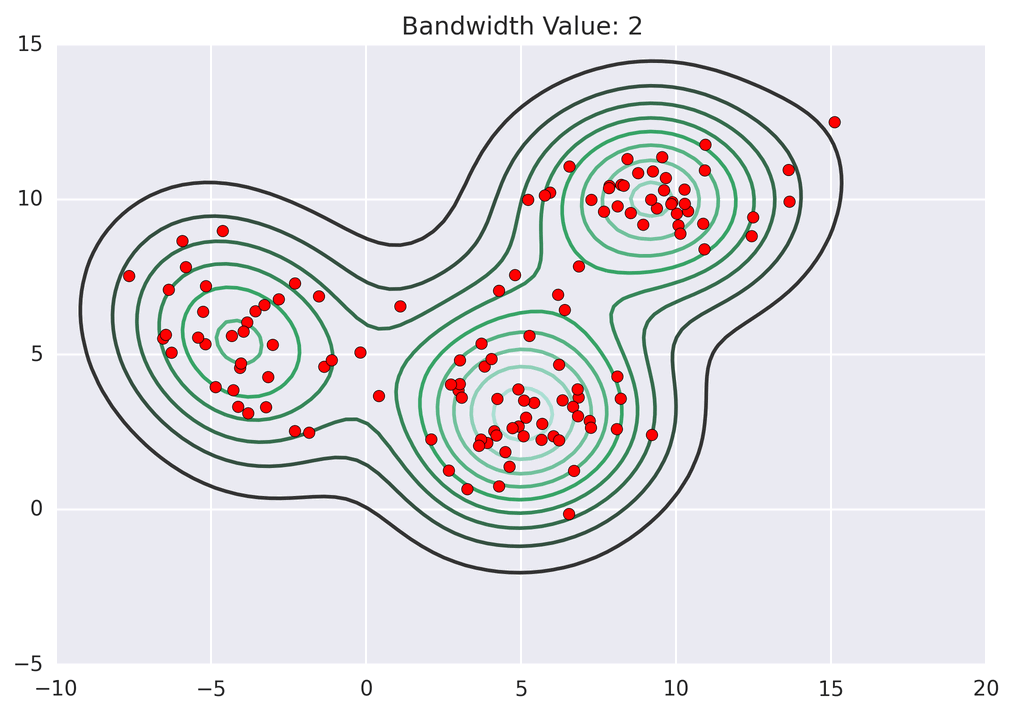Image: source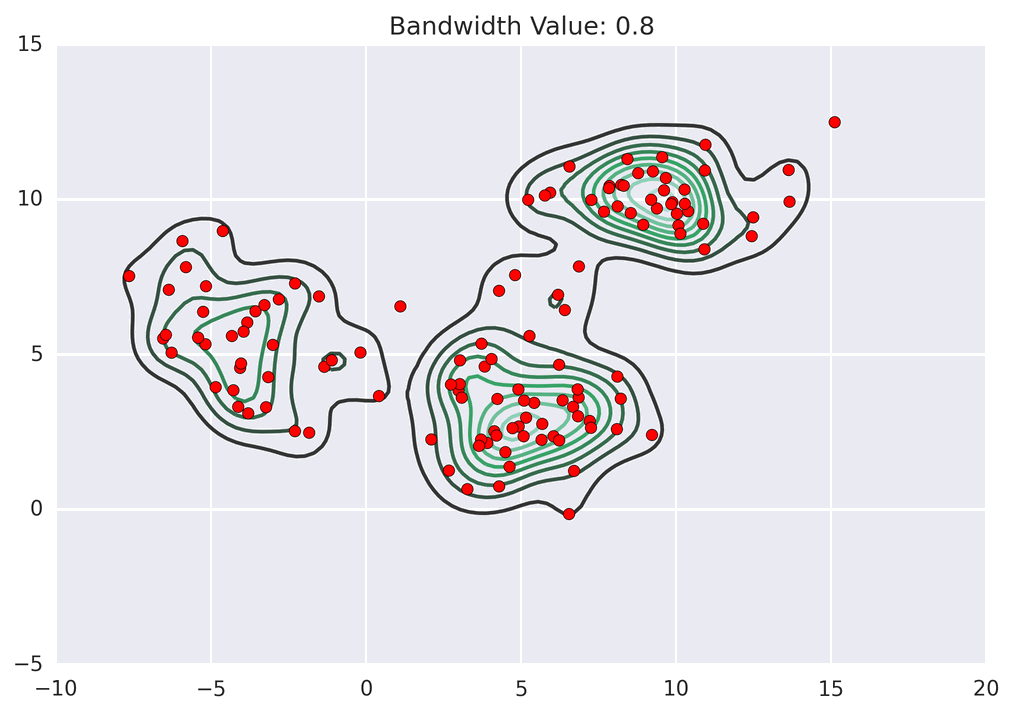Image: source

Here, bandwidth (or kernel bandwidth) is the size of the region/window used to calculate the mean of the data points.

`from sklearn.cluster import MeanShift, estimate_bandwidthbandwidth = estimate_bandwidth(X, quantile=0.2, n_samples=len(X))ms = MeanShift(bandwidth=bandwidth, bin_seeding=True)y_ms = ms.fit_predict(X)print ("Bandwidth value: " + str(bandwidth))print ("Number of predicted clusters: " + str(len(ms.cluster_centers_)))`

Visualizing the clusters:

# 5. Spectral Clustering

In spectral clustering, the input data points are treated as nodes of a graph. Two nodes are connected if the data points are similar and thus different groups/clusters are formed based on similarity of the points. Points in two clusters are dissimilar than each other.

`from sklearn.cluster import SpectralClusteringsp_cls = SpectralClustering(n_clusters = 3, random_state=0)y_sp_cls = sp_cls.fit_predict(X)`

Visualizing the clusters:

# 6. Density-Based Spatial Clustering of Applications with Noise (DBSCAN)

Given a set of points in some space, it groups together points that are closely packed together (points with many nearby neighbors), marking as outliers points that lie alone in low-density regions (whose nearest neighbors are too far away).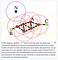Image: source
`from sklearn.cluster import DBSCAN# metric to use when calculating distance between # instances in a feature arraydbscan = DBSCAN(eps=0.5, metric='euclidean')y_dbscan = dbscan.fit_predict(X)labels = dbscan.labels_# Number of clusters in labels, ignoring noise if present.n_clusters_ = len(set(labels)) - (1 if -1 in labels else 0)print ("Number of predicted clusters: " + str(n_clusters_))`

Visualizing the clusters:

# 7. Ordering Points To Identify the Clustering Structure (OPTICS)

This is another form of density based clustering and closely related to DBSCAN. However overcomes one of the DBSCAN’s disadvantages, i.e. inability of detecting clusters of variable data densities. Clusters are then extracted using a DBSCAN-like method (cluster_method = ‘dbscan’) or an automatic technique (cluster_method = ‘xi’).

Density based clustering algorithms work well when datasets have densities and drops in-between the clusters.

Couple of advantages of DBSCAN and OPTICS:

• We don’t need to specify the number of clusters before-hand.
• Outliers or noise can be identified.
`from sklearn.cluster import OPTICS# cluster_method : The extraction method used to extract # clusters using the calculated reachability and ordering. # Possible values are "xi" and "dbscan".opt = OPTICS(min_samples=50, xi=.05, \             min_cluster_size=.05, cluster_method='xi', \             metric='minkowski', algorithm = 'auto')y_opt = opt.fit_predict(X)`

Visualizing the clusters:

# 8. Balanced Iterative Reducing and Clustering using Hierarchies (BIRCH)

This is a hierarchical clustering approach and suitable for very large datasets. This algorithm uses an in memory data structure called clustering feature (CF) tree. Refer here for further reading.

`from sklearn.cluster import Birchbr = Birch(n_clusters=3)y_br = br.fit_predict(X)`

Visualizing the clusters:

# Plotting based on actual data

Now let’s see, if we plot a scatter plot based on actual data how does it look like!

# Conclusion

Scikit Learn is a single-node framework and contains various effective tools for predictive data analysis. This works well for limited data. In case we need to work with massive amount of data, we can try Apache Spark MLlib.

Thanks for reading!! If you have enjoyed, Clap & Share it!! To see similar posts, follow me on Medium & LinkedIn.

Tech enthusiast, Azure Big Data Architect.

## More from Prosenjit Chakraborty

Tech enthusiast, Azure Big Data Architect.